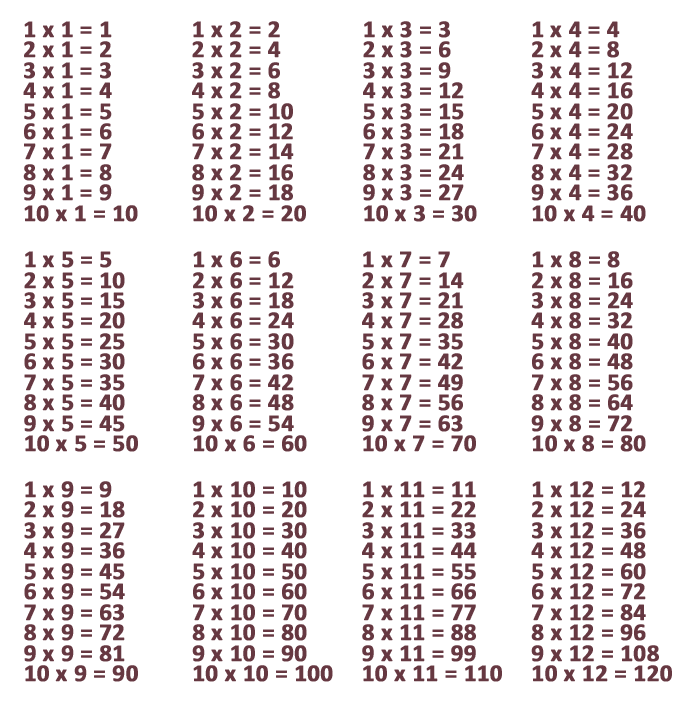Once the math basics, such as adding and subtracting, have been adequately learned, the student can start learning multiplication tables. These form the foundation of virtually all arithmetic operations in higher grades, which is why it is so important for children to know the tables well. But what’s the best way to practice these multiplication tables? Children learn best if they do so in a way that’s fun for them. And who doesn’t like playing games? That’s why at MultiplicationGames.com kids can learn math with the help of multiplication games. We have tables in all degrees of difficulty as you can see on the overview page, so there is sure to be a game for every child that he or she will enjoy.

What exactly is a multiplication table?

In brief, a multiplication table is the number of times you add up the same number. In the example below we use the 5 times table. For example, if you have 3 times the number 5, then the associated equation is 3 x 5 with an outcome of 15 (5 + 5 + 5). So the easiest way to work out a multiplication table is to calculate it back to the addition. If you have the equation 3 x 5, then you know that this is equal to: 5 + 5 + 5. If you practice frequently, at a given moment you will know the answers to the multiplication tables by heart and will be able to complete all of them. You will then be able to multiply much faster, especially if you are dealing with larger numbers.

Why do I have to know the multiplication tables?

You will have to use the skill of multiplication a lot both in school and for the rest of your life. If you work in a store, for example, and someone orders several of the same items, you will have to calculate how much these items cost all together with the help of multiplication, as you will have to multiply the number of items by the price. Or imagine you’re working in your yard and want to build a patio. You will use the multiplication tables to calculate how many stones you will need for this patio. If you keep having to calculate it back to the additions as we previously described on this page, you will lose a lot of valuable time. Instead, if you can use your memorized multiplication tables you will not only count faster, the chance of making a mathematical error is also a lot smaller.

Which multiplication tables are the most important?The multiplication tables you will encounter most often in life are multiplication tables 1-12, which is why these are taught in all schools. These usually go to 10, for example: 1 x 12, 2 x 12, 3 x 12 etc., up to 10 x 12. Most multiplication games on this website go up to the 12 times table, and often the game allows you to choose exactly which table you want to practice.

Why not do math using a calculator?

Many children prefer to use a calculator because this is a lot faster. But if you need to pull out the calculator for every simple math problem, you’ll lose a lot of time. What’s more, people will also find it quite strange if you need a calculator for every simple math problem. If you are able to quickly solve lots of multiplication equations, you will save a lot of time later on in life. But the most obvious reason for not solving multiplication tables using a calculator is because you aren’t allowed to use a calculator during most exams! So if you don’t know the multiplication tables by heart, you’ll never be able to pass an exam. That alone is a good enough reason to use our multiplication games. And be honest: Is it really so terrible to play games while learning something?